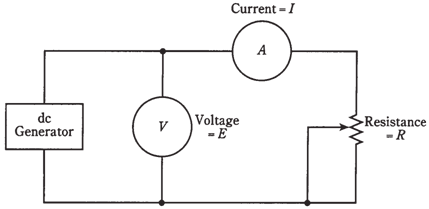## Voltage calculations Assignment Help

Assignment Help: >> Electrical Engineering - Voltage calculations

Voltage  calculations

The 2nd use of Ohm's Law is to find the unknown voltages when the current and the resistance are known to us. For problems given below, uncover the ammeter and cover the voltmeter scale instead in the mind.Problem 1:

Suppose the potentiometer is set to 100 ohms, and the measured current is

10 mA. What is the dc voltage?

Use the formula E = I R. First, convert the current to amperes: 10 mA = 0. 01 A. Then multiply: E = 0. 01 * 100 = 1V. That is a low, safe voltage, a bit less than what is produced  by a flashlight cell.

Problem 2:

Adjust potentiometer to the value of 157 K  , and let the current reading be 17 mA. What is voltage of the source?

Now you have to convert the resistance and the current both values to their original units. A resistance of 157 K is 157,000 and a current of 17 mA is 0.017 A. Then E = IR = 0.017 * 157,000 = 2669V = 2.669 kV. You may have desire to round this off to 2.67 kV. This is a dangerous voltage. If you touch the terminals you will get clobbered.

It is not a good idea to specify your answer to a problem with significant figures than you are given. The engineers and scientists follow the rule of significant figures: keep to the least number of digits given in the data.